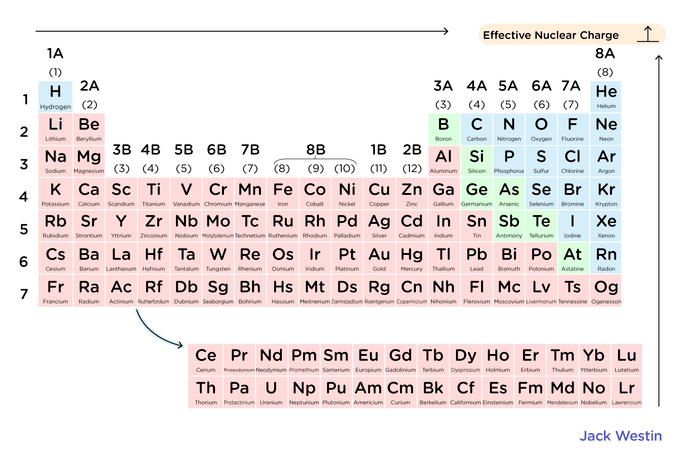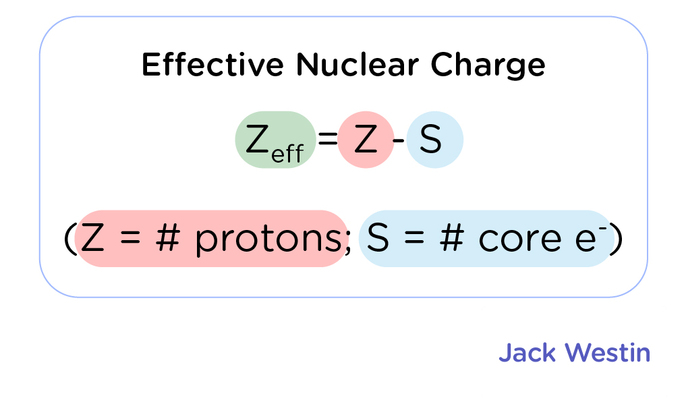Complimentary 1-hour tutoring consultation

MCAT Content / Electronic Structure / Effective Nuclear Charge

### Effective nuclear charge

Topic: Electronic Structure

The effective nuclear charge is the net positive charge experienced by an electron in a multi-electron atom.

Electrons in an atom can shield each other from the pull of the nucleus. This effect, called the shielding effect, describes the decrease in the attraction between an electron and the nucleus in any atom with more than one electron shell. The more electron shells there are, the greater the shielding effect experienced by the outermost electrons.The magnitude of the shielding effect is difficult to calculate precisely. As an approximation, we can estimate the effective nuclear charge on each electron.

The effective nuclear charge (often symbolized as Zeff or Z*) is the net positive charge experienced by an electron in a multi-electron atom.The effective nuclear charge on an electron is given by the following equation:

Zeff = Z – S

where Z is the number of protons in the nucleus (atomic number), and S is the number of electrons between the nucleus and the electron in question (the number of non-valence electrons).

Consider a neutral neon atom (Ne), a sodium cation (Na+), and a fluorine anion (F). All have 2 non-valence electron, while Ne has 10 protons, Na has 11 and F has 9.

• Zeff(Ne) = 10 – 2 = 8+
• Zeff(F) = 9 – 2 = 7+
• Zeff(Na+) = 11 – 10 = 1+Key Points

• The shielding effect describes the balance between the pull of the protons on valence electrons and the repulsion forces from inner electrons.

• The shielding effect explains why valence-shell electrons are more easily removed from the atom.

• The effective nuclear charge is the net positive charge experienced by valence electrons. It can be approximated by the equation: Zeff = Z – S, where Z is the atomic number and S is the number of shielding electrons.

Key Terms

valence shell: is the outermost shell of every element.

nucleus: the positively charged central part of an atom, made up of protons and neutrons

electron: each electron has a negative charge (-1) with weight so small it’s normally negligible as compared to proton or neutron.

shielding effect: the attraction between an electron and the nucleus which is reduced by inner electrons

effective nuclear charge: the net positive charge experienced by an electron

Billing Information